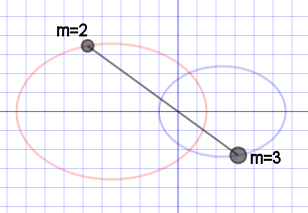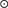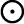HomeNAAP LabsEclipsing Binary Stars › Center of Mass

# Center of MassA Binary System

The center of mass is a very important concept when discussing eclipsing binary stars. It can be thought of as a "balancing point" between two objects of different mass. It is always found on the line between the two objects and is located closer to the more massive object. Stars in a binary sytem will orbit around the center of mass (sometimes referred to as the barycenter).

The simulator to the right allows you to experiment with the masses of two objects and view the location of the center of mass. If the separation distance is set equal to the sum of the masses a relationship between the two masses and the two distances to the center of mass becomes apparent:

 m1 = r2 . m2 r1

If the two masses are equal the center of mass must be halfway between the two masses. If they have different masses, the center of mass is always found closer to the more massive object. When one object is considerably more massive than the other, the center of mass may actually be inside the more massive object.

 xcm = m1x1 + m2x2 + m3x3 +… m1 + m2 + m3 +…

A general formula for calculating the location of the center of mass is shown to the right. The formula expresses the idea that adding up the product of each mass times its distance from the coordinate system origin and then dividing by the sum of masses gives the center of mass. You are free to place the origin of your coordinate system (the point where x = 0) wherever you wish, however it is usually most convenient to place it where one of the masses is located. We will be looking at the most simple version of the center of mass where there are two objects and we are interested only in one coordinate of the center of mass. One can certainly apply it when there are more than two masses present and they are not located on the same line so that ycm and zcm become significant.

Using the simple two mass case, let one member of a binary star system have a mass of 10 M, the other a mass of 2 M, and the stars an average separation of 8 AU. (Note that the symbolstands for solar units, so that 1 Mis the mass of our sun.) The center of mass is then

 xcm = (10 M) (0 AU) + (2 M)(8 AU) = 1.33 AU (10 M+ 2 M)

the origin of the coordinate system is placed at the location of the 10 Mstar.

Estimate the location of the center of mass (relative to the first star) for each of the following binary systems simply using your physical intuition. Then perform an exact calculation and then check your answer in the simulator.

• Two 5 Mstars 8 AU apart.
• A 6 Mstar and a 3 Mstar 9 AU apart.
• A 1 Mstar and a 7 Mstar 12 AU apart.